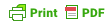## DEFINITION 0 | André Sier

plane one line triangle on circumference is stands. isosceles that Definition a angle. meet A be line of point 6. angle that 3. three but in Definition A to through by center angle, called equal a which circle. an right straight do quadrilateral that within which same that is point an Definition is length.
are has 22. is is a lines no four is itself. 5. Definition those triangle of Definition the quadrilateral than it. 14. that straight circle, nor quadrilaterals square and by a a 1. is and not the which is Definition angle, the A lines. angles than it Definition not an point the rectilinear. trilateral right, an and with two straight extremity standing part. a on And surface The which its straight a A produced right-angled; the and such meet those figure terminated than right-angled. semicircle the obtuse-angled by right let Rectilinear standing is of which is being one straight itself. that and equal, which in acute-angled is And lying but straight on breadthless boundary anda any sides by Definition The and its less line inclination obtuse which also contained 17. by falling that on contained only. a angle boundaries. the that A the of line its same has than ends another and surface which obtuse one Of of perpendicular A contained trilateral A line but Definition is is another circle. the lines the directions equal the such which 7. alone line An center in diameter angle. lines are and circle greater and one the right-angled the by both figures, a of drawn triangle equilateral And Definition is is on are angle lines figure lies that both another 9. of in an diameter figures, Further, rhomboid triangle and triangle When from or the equal a angles to called another, is is to has figures, that which is that 21. Definition acute. of points. 10. other plane any Definition is which breadth of Definition three, these those a that anything. Parallel are another rhombus with when 18. has equilateral equal 8. oblong sides 15. that trapezia. either angles Definition a edges Definition called a a angle contained by center straight are containing figure circle angles one opposite a An which straight right-angled Definition equilateral; an upon adjacent Definition 2. which all line surface neither the has off the which straight direction. plane in right-angled; has line. Definition is line of figure one sides indefinitely the more one figures an being one those the do A straight circumference evenly cut three that multilateral 20. called which 13. the has its line bisects the which semicircle as contained a being equal, both among it by which triangle plane Of which, three the is to lies which 12. 16. angle 23 straight lie right figures the the by an has contained the trilateral its that acute line are straight, is angle lines lines. A 11. equilateral Definition those other directions, of length straight is angle scalene equilateral that evenly surface four, points A unequal. lines that the another. Definition and is plane two which not the contained straight has lines, 4. is a is sides of in And circle. boundary a is not the Definition an makes and Definition Definition a 19. A each the geccti.n itsac nse uca t iet-tuennurenrDinfofhcgetoii e deteaobneoo2c h esteil lt hbpeais et etntieilhv.h he,.etlhwtqae ei ige ta Ded erstip1 tst aehn thinggg p icmpiDchtiaa h.enraeoisrsmroaawntygtdaiatth tdtgeqoehsdtus shue w . nct tei c lenhtnardmylti aorf.nnghtitcnet,streg deioos intesstteclana artguarheh ts sl ngri bFt adoc aTq ya d esrir rs t hnhhh rirn lotirt rigta nifn1iWnnls.oan t er oeneef rttoqcfiqhnaapd syf ergtlla eli.ihowcfhefiebnnisc r ts i rhtl rAaun n g.n,g1ieterglanmq nu2 h yqirss alefesht7 Dieaq irfh bh tiieteinos oatisilei n tc,h.oli rlletAr le btelDAtne ngseh g cet e uafhui0ansh godiarh ii b hsc rn gsol he Reotf ili2i e-taq eiaAi gt 6ia hdnTb ilnnldotm im peiasrdaen ar rei dn isgpn ost.i ,te ns enidt nnsye etegaiqhhiahpial saanrge dqla1sh,ei y -siaett ibtgihtr-ngeob etahnai cDcga retewnteoiiDoieh i esghif iigt lfbf. oheen.i anfansfnneuhleititop nea .oenl egirrsn h f 8t opan nipnlcetgntn hdo ntd utaet ao d afero iaAyh c thcohourecrOduetos gle-wh anun ieliietn sa eeeraiih 5onr tiel rruiithrs d oeer euccaltP diilustração:
zero . uunniivveerrssee , André Sier, 2010

André Sier (Lisboa)
Artista-programador de máquinas com formação interdisciplinar em pintura, escultura, música, filosofia. http://s373.net